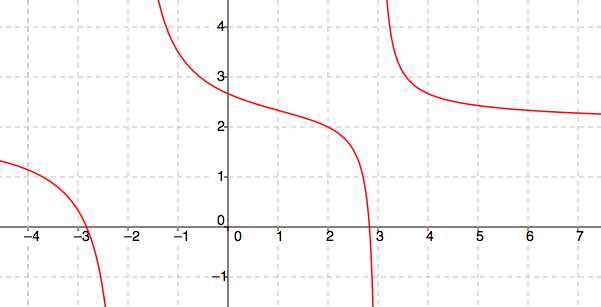### Home > PC > Chapter 10 > Lesson 10.1.1 > Problem10-11

10-11.

Find all asymptotes and holes for the function $f(x) = \frac { 2 x ^ { 2 } - 16 } { x ^ { 2 } - x - 6 }$.

Start by factoring the numerator and denominator and simplifying.

Vertical asymptotes and holes occur when the denominator is equal to $0$.

Horizontal asymptotes are found by considering what happens to the function as $x → ±∞$.

Identify the dominant terms and simplify.